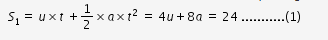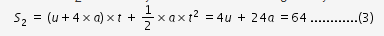# A body moving with uniform acceleration travels a distance of 24m and 64m

A body moving with uniform acceleration travels a distance of 24m and 64m rurind the first two equal consecutive intervals of time each of duration 4 seconds.

Determine the initial velocity and acceleration of moving body.

let initial velocity u m/s and acceleration a m/s2 .
let distance travelled in first 4 s interval is S1., it is given by,from (1), we get, u+2×a = 6 …(2)
after 4 sec, velocity = u+a×t = u+4a
distance S2 travelled by second 4 s interval is given byfrom (3), we get u +6×a = 16 …(4)
By solving (2) and (4), we get u = 1 m/s , a = 2.5 m/s2